﻿ 一种新的防电击保护自动检测装置的研制

# 一种新的防电击保护自动检测装置的研制A New Kind of Auto Testing Device Developed for Protection against Electric Shock

Abstract: In this paper, a new anti-shock protection automatic detection device is developed. When detecting, the detection device automatically realizes peak voltage off and releases residual energy through the discharge load. The system simultaneously collects discharge voltage and current, calculating residual energy. The detection device adopts the microcontroller STM32F407 as the control core, which is used to realize the initialization of the AD7606 liquid crystal display and the USB interface, the detection control and the acquisition, storage and data operation processing of the relevant measured data. The paper gives the circuit diagram and waveform diagram.

1. 引言

2. 国内外研究情况

3. 工作原理

4. 装置组成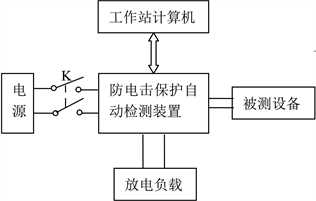Figure 1. Design diagram of auto testing device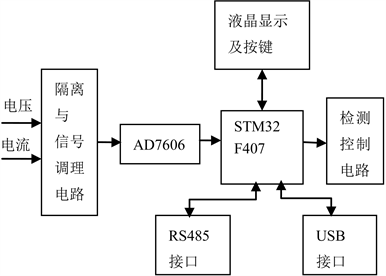Figure 2. Frequency measurement system

4.1. 隔离与信号调理电路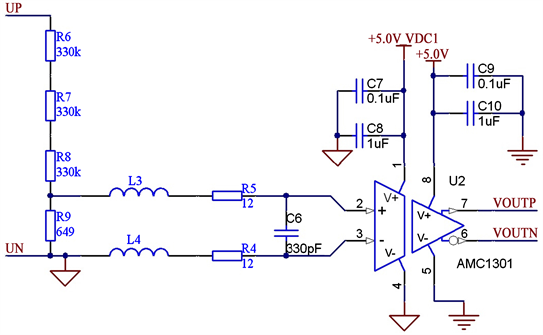Figure 3. Voltage channel based on AMC1301

4.2. 电压调理电路

${U}_{peak}=0.25×\frac{{R}_{6}+{R}_{7}+{R}_{8}+{R}_{9}}{{R}_{9}}=381.6\text{\hspace{0.17em}}\text{V}$ (1)

4.3. 电流调理电路

${U}_{peak}=\frac{0.25}{{R}_{1}}=5\text{\hspace{0.17em}}\text{A}$ (2)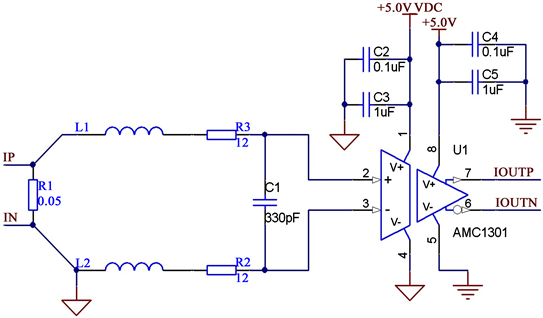Figure 4. Current channel based on AMC1301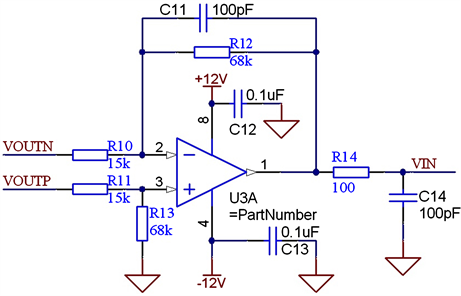Figure 5. Signal conditioning circuit

${k}_{v}=\frac{{R}_{9}}{{R}_{6}+{R}_{7}+{R}_{8}+{R}_{9}}×8.2×\frac{{R}_{12}}{{R}_{10}}=0.02435$ (3)

${k}_{i}={R}_{1}×8.2×\frac{{R}_{12}}{{R}_{10}}=1.8587$ (4)

5. 仿真

5.1. 仿真电路图

5.2. 仿真波形

5.3. 放电过程能量计算

5.3.1. 理论计算值

$\begin{array}{c}{E}_{理论}=0.5{C}_{1}{U}^{2}+0.5{C}_{2}{U}^{2}\\ =0.5×0.47×{10}^{-6}×{310.9998}^{2}\text{\hspace{0.17em}}\text{J}+0.5×4.7×{10}^{-6}×{310.9998}^{2}\text{\hspace{0.17em}}\text{J}\\ =250.0232\text{\hspace{0.17em}}\text{mJ}\end{array}$

5.3.2. 矩形法计算

$\begin{array}{c}{E}_{计算}=\frac{\Delta t}{R}×\left[\underset{i=1}{\overset{n}{\sum }}{U}_{i}^{2}\right]\\ =\frac{6.066×{10}^{-6}}{2000}×\left({310.9998}^{2}+{310.8474}^{2}+{310.7041}^{2}+\cdots \right)\\ =250.6792\text{\hspace{0.17em}}\text{mJ}\end{array}$

5.3.3. 梯形法计算

$\begin{array}{c}{E}_{计算}=\frac{\Delta t}{2R}×\left[{U}_{1}^{2}+2\underset{i=2}{\overset{n}{\sum }}{U}_{i}^{2}\right]\\ =\frac{6.066×{10}^{-6}}{2×2000}×\left[{310.9998}^{2}+2×\left({310.8474}^{2}+{310.7041}^{2}+{310.5560}^{2}+\cdots \right)\right]\\ =250.5254\text{\hspace{0.17em}}\text{mJ}\end{array}$

5.3.4. 辛普森法计算

$\begin{array}{c}{E}_{计算}=\frac{\Delta t}{3R}×\left[{U}_{1}^{2}+4\underset{i=1}{\overset{n}{\sum }}{U}_{2i}^{2}+2\underset{i=1}{\overset{n}{\sum }}{U}_{2i+1}^{2}\right]\\ =\frac{6.066×{10}^{-6}}{3×2000}×\left[{310.9998}^{2}+4×\left({310.8474}^{2}+{310.5560}^{2}+\cdots \right)\\ \text{\hspace{0.17em}}\text{\hspace{0.17em}}+2×\left({310.7041}^{2}+{310.4084}^{2}+\cdots \right)\right]\\ =250.5253\text{\hspace{0.17em}}\text{mJ}\end{array}$

5.3.5. 科特斯法计算

$\begin{array}{c}{E}_{计算}=\frac{2\Delta t}{45R}×\left[7{U}_{1}^{2}+32\underset{i=0}{\overset{n}{\sum }}{U}_{4i+2}^{2}+12\underset{i=0}{\overset{n}{\sum }}{U}_{4i+3}^{2}+32\underset{i=0}{\overset{n}{\sum }}{U}_{4i+4}^{2}+14\underset{i=0}{\overset{n}{\sum }}{U}_{4i+5}^{2}\right]\\ =\frac{2×6.066×{10}^{-6}}{45×2000}×\left[7×{310.9998}^{2}+32×\left({310.8474}^{2}+{310.2607}^{2}+\cdots \right)\\ \text{\hspace{0.17em}}\text{\hspace{0.17em}}+12×\left({310.7041}^{2}+{310.1131}^{2}+\cdots \right)+32×\left({310.5560}^{2}+{309.9645}^{2}+\cdots \right)\\ \text{\hspace{0.17em}}\text{\hspace{0.17em}}+14×\left({310.4084}^{2}+{309.8182}^{2}+\cdots \right)\right]\\ =250.5253\text{\hspace{0.17em}}\text{mJ}\end{array}$

5.3.6. 误差计算

6. 结论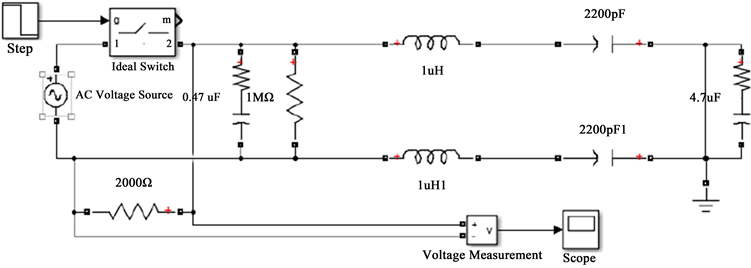Figure 6. Simulation circuit diagram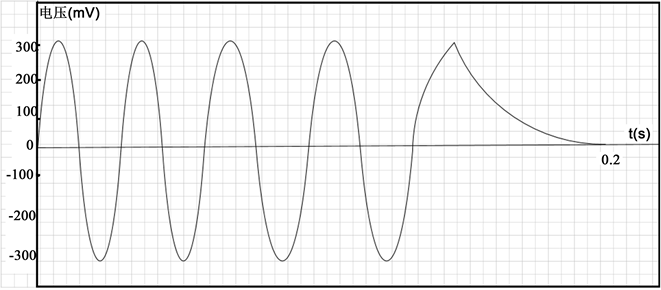Figure 7. Simulation waveform diagram

 徐正明, 石晓晶. 峰值检测示波器的设计[J]. 仪表技术与传感器, 2014(4): 14-15, 24.

 范兵兵, 艾信友, 曾研, 胡凯旋, 任泽明. 基于STM32的位置定位系统设计[J]. 科技创新与应用, 2018(12): 90-91.

 颜培玉, 段瑞彬, 刘文吉, 唐德渝, 王克宽. 基于AD7606的焊接电弧电信号高精度数据采集系统设计[J]. 热加工工艺, 2018(17): 176-179.

 张文忠, 刘继广, 林联君, 邵秀稳, 邓振进, 邱栋梁. IEC 61010-1: 2010体外诊断设备电击防护要求分析[J]. 医疗卫生装备, 2017, 38(11): 79-82.

 刘继广, 王伟, 邓振进, 徐勤, 尹勇. 医用电气设备可触及金属部件保护接地要求浅析[J]. 医疗卫生装备, 2017, 38(2): 95-97.

Top